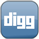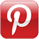The other day we looked at what was necessary to draw lines with canvas. First we covered setting the line style, then we looked at drawing the actual lines. What we were using in those examples were solid colors. What if we wanted to use a gradient instead? Here's how.

Before we can draw with a gradient, we need to create it. We can do this by using the createLinearGradient() method of the context object. This method takes four parameters, the starting point(x1, y1) and the ending point(x2, y2) of the gradient. See below.

``` var context; var gradient; context = canvasTag.getContext('2d'); gradient = context.createLinearGradient(0, 0, 100, 100); ```

Once we've created our gradient, we need to define what colors we'll be using. We can do this by adding color stops to our gradient object. We can do this by using the addColorStop() method. This method takes two parameters-the stop- a value between 0 and 1 that represents the position of the gradient color, and the second parameter is the color itself. Let's take a look.

``` var context; var gradient; context = canvasTag.getContext('2d'); gradient = context.createLinearGradient(0, 0, 100, 100); gradient.addColorStop(0, "yellow"); gradient.addColorStop(1, "red"); ```

Lastly, we come to setting the gradient that we've created. We can do this by using the strokeStyle property of the context object. In the past, we've set this to a solid color-but we can also set it to a gradient object. Let's put it all together.

``` var context; var gradient; context = canvasTag.getContext('2d'); gradient = context.createLinearGradient(0, 0, 100, 100); gradient.addColorStop(0, "yellow"); gradient.addColorStop(1, "red"); context.strokeStyle = gradient; context.lineWidth = 10; context.beginPath(); context.moveTo(25, 25); context.lineTo(75, 75); context.stroke(); context.closePath(); ```

Your browser does not support the canvas tag.